×#### Thank you for registering.

One of our academic counsellors will contact you within 1 working day.

Click to Chat

1800-1023-196

+91-120-4616500

CART 0

• 0

MY CART (5)

Use Coupon: CART20 and get 20% off on all online Study Material

ITEM
DETAILS
MRP
DISCOUNT
FINAL PRICE
Total Price: Rs.

There are no items in this cart.
Continue Shopping• Complete JEE Main/Advanced Course and Test Series
• OFFERED PRICE: Rs. 15,900
• View Details

```Chapter 7: Statistics Exercise – 7.4

Question: 1

Following are the lives in hours of 15 pieces of the components of aircraft engine. Find the median: 715, 724, 725, 710, 729, 745, 694, 699, 696, 712, 734, 728, 716, 705, 719.

Solution:

Lives in hours of is pieces are = 715, 724, 725, 710, 729, 745, 694, 699, 696, 712, 734, 728, 716, 705, 719

Arrange the above data in ascending order = 694, 696, 699, 705, 710, 712, 715, 716, 719, 721, 725, 728, 729, 734, 745

N = 15 (odd)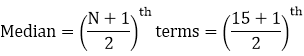terms = 8th terms = 716

Question: 2

The following is the distribution of height of students of a certain class in a certain city:

Height (in cm):
160 - 162
163 - 165
166 - 168
169 - 171
172 - 174

No of students:
15
118
142
127
18

Find the median height.

Solution:

Class interval (exclusive)
Class interval  (inclusive)
Class interval frequency
Cumulative frequency

160 – 162
159.5 – 162.5
15
15

163 – 165
162.5 – 165.5
118
133 (F)

166 – 168
165.5 – 168.5
142 (f)
275

169 – 171
168.5 – 171.5
127
402

172 – 174
171.5 – 174.5
18
420

N = 420

We have N = 420,

N/2 = 420/ 2 = 120

The cumulative frequency just greater than N/2 is 275 then 165.5 - 168.5 is the median class such, that

L = 165.5, f = 142, F = 133 and h = 168.5 - 165.5 = 3= 165.5 – 0.27

= 165.23

Question: 3

Following is the distribution of I.Q of 100 students. Find the median I.Q.

I.Q:
55 - 64
65 - 74
75 - 84
85 - 94
95 - 104
105 - 114
115 - 124
125 - 134
135 - 144

No of students:
1
2
9
22
33
22
8
2
1

Solution:

Class interval (exclusive)
Class interval  (inclusive)
Class interval frequency
Cumulative frequency

55 – 64
54.5 – 64-5
1
1

65 – 74
64.5 – 74.5
2
3

75 – 84
74.5 – 84.5
9
12

85 – 94
84.5 – 94.5
22
34 (f)

95 – 104
94.5 – 104.5
33 (f)
67

105 – 114
104.5 – 114.5
22
89

115 – 124
114.5 – 124.5
8
97

125 – 134
124.5 – 134.5
2
99

135 – 144
134.5 – 144.5
1
100

N = 100

We have N = 100 N/ 2 = 100/ 2 = 50

The cumulative frequency just greater than N/ 2 is 67 then the median class is 94.5 - 104.5 such that L = 94.5, F = 33, h = 104.5 - 94.5 = 10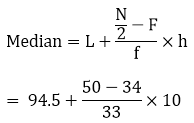= 94.5 + 4.88

= 99.35

Question: 4

Calculate the median from the following data:

Rent (in Rs):
15 - 25
25 - 35
35 - 45
45 - 55
55 - 65
65 - 75
75 - 85
85 - 95

No of houses:
8
10
15
25
40
20
15
7

Solution:

Class interval
Frequency
Cumulative frequency

15 – 25
8
8

25 – 35
10
18

35 – 45
15
33(f)

45 – 55
25
58

55 – 65
40(f)
28

65 – 75
20
38

75 – 85
15
183

85 – 95
7
140

N = 140

We have N = 140 N/ 2 = 140/ 2 = 70

The cumulative frequency just greater than N/ 2 is 98 then median class is 55 - 65 such that L = 55, f = 40, F = 58, h = 65 - 55 = 10= 55 + 3 = 58

Question: 5

Calculate the median from the following data:

Marks below:
10 – 20
20 - 30
30 - 40
40 - 50
50 - 60
60 - 70
70 - 80
85 - 95

No of students:
15
35
60
84
96
127
198
250

Solution:

Marks below
No of students
Class interval
Frequency
Cumulative frequency

10
15
0 – 10
15
15

20
35
10 – 20
20
35

30
60
20 – 30
25
60

40
84
30 – 40
24
84

50
96
40 – 50
12
96(F)

60
127
50 – 60
31 (f)
127

70
198
60 – 70
71
198

80
250
70 – 80
52
250

N = 250

We have N = 250, N/ 2 = 250/ 2 = 125

The cumulative frequency just greater than N/ 2 is 127 then median class is 50 – 60 such that L = 50, f = 31, F = 96, h = 60 -50 = 10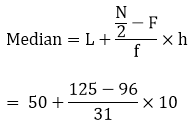= 50 + 9.35

= 59.35

Question: 6

Calculate the missing frequency from the following distribution, it being given that the median of the distribution is 24.

Age in years:
0 - 10
10 – 20
20 - 30
30 - 40
40 - 50

No of persons:
5
25
?
18
7

Solution:

Class interval
Frequency
Cumulative frequency

0 – 10
5
5

10 – 20
25
30 (F)

20 – 30
x (f)
30 + x

30 – 40
18
48 + x

40 – 50
7
55 + x

N = 170

Given Median = 24 Then, median class = 20 - 30 L = 20,   h = 30 -20 = 10, f = x,   F = 30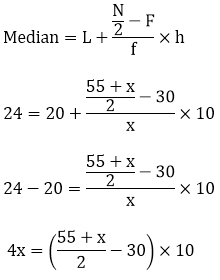4x = 275 + 5x - 300

4x - 5x = – 25

- x = – 25

x = 25

Missing frequency = 25

Question: 7

The following table gives the frequency distribution of married women by age at marriage.

Age (in years)
Frequency
Age (in years)
Frequency

15 – 19
53
40 – 44
9

20 – 24
140
45 – 49
5

25 – 29
98
50 – 54
3

30 – 34
32
55 – 59
3

35 – 39
12
60 and above
2

Calculate the median and interpret the results

Solution:

Class interval (exclusive)
Class interval (inclusive)
Frequency
Cumulative frequency

15 – 19
14.5 – 19.5
53
53 (F)

20 – 24
19.5 – 24.5
140 (f)
193

25 – 29
24.5 – 29.5
98
291

30 – 34
29.5 – 34.5
32
323

35 – 39
34.5 – 39.5
12
335

40 – 44
39.5 – 44.5
9
344

45 – 49
44.5 – 49.5
5
349

50 – 54
49.5 – 54.5
3
352

55 - 54
54.5 – 59.5
3
355

60 and above
59.5 and above
2
357

N = 357

N = 357 N/2 = 357/2 = 178.5 The cumulative frequency just greater than N/2 is 193,

Then the median class is 19.5 – 24.5 such that l = 19.5, f = 140, F = 53, h = 25.5 – 19.5 = 5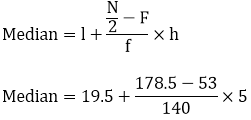Median = 23.98, Nearly half the women were married between the ages of 15 and 25

Question: 8

The following table gives the distribution of the life time of 400 neon lamps:

Life time:
Number of lamps

1500 - 2000
14

2000 - 2500
56

2500 – 3000
60

3000 – 3500
86

3500 – 4000
74

4000 – 4500
62

4500 – 5000
48

Find the median life.

Solution:

We can find cumulative frequencies with their respective class intervals as below:

Life time
Number of lamps fi
Cumulative frequency (cf)

1500 – 2000
14
14

2000 – 2500
56
70

2500 – 3000
60
130

3000 – 3500
86
216

3500 – 4000
74
290

4000 – 4500
62
352

4500 – 5000
48
400

Total (n)
400

Now we may observe that cumulative frequency just greater than n/2 (400/2 = 200) is 216 belongs to class interval 3000 - 3500 Median class = 3000 - 3500

Lower limits (l) of median class = 3000 Frequency (f) of median class = 86

Cumulative frequency (cf) of class preceding median class = 130 Class size (h) = 500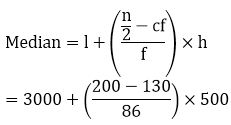= 3000 + (35000/86) = 3406.98 So, median life time of lamps is 3406.98 hours

Question: 9

The distribution below gives the weight of 30 students in a class. Find the median weight of students:

Weight (in kg):
40 - 45
45 - 50
50 - 55
55 - 60
60 - 65
65  - 70
70 - 75

No of students:
2
3
8
6
6
3
2

Solution:

We may find cumulative frequency with their respective class intervals as below:

Weight (in kg)
Number of students fi
Cumulative frequency (cf)

40 – 45
2
2

45 – 50
3
5

50 – 55
8
13

55 – 60
6
19

60 – 65
6
25

65 – 70
3
28

70 – 75
2
30

Cumulative frequency just greater than n/ 2 (i.e. 30/ 2 = 15) is 19, belonging to class interval 55 – 60 Median class = 55 – 60 Lower limit (l) of median class = 55 Frequency (f) of median class = 6 Cumulative frequency (cf) = 13 Class size (h) = 5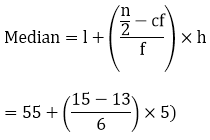= 55 + 10/6 = 56.666

So, median weight is 56.67 kg

Question: 10

Find the missing frequencies and the median for the following distribution if the mean is 1.46

No of accidents:
0
1
2
3
4
5
Total

Frequencies (no of days):
46
?
?
25
10
5
200

Solution:

No of accidents (x)
No of days (f)
fx

0
46
0

1
x
x

2
y
2y

3
25
75

4
10
40

5
5
25

N = 200
Sum = x + 2y + 140

Given N = 200 46 + x + y + 25 + 10 + 5 = 200 x + y = 200 – 46 – 25 – 10 – 5 x + y = 114  ---- (1)

And, Mean = 1.46 Sum/ N = 1.46 (x + 2y + 140)/ 200 = 1.46 x + 2y = 292 – 140 x + 2y = 152 ---- (2)

Subtract equation (1) from equation (2) x + 2y – x – y = 152 – 114 y = 38

Putting the value of y in equation (1), we have x = 114 – 38 = 76

No of accidents
No of days
Cumulative frequency

0
46
46

1
76
122

2
38
160

3
25
185

4
10
195

5
5
200

N = 200

We have, N = 200 N/2 = 200/2 = 100 The cumulative frequency just more than N/2 is 122 then the median is 1

Question: 11

An incomplete distribution is given below:

Variable:
10 – 20
20 - 30
30 - 40
40 - 50
50 - 60
60 - 70
70 - 80

Frequency:
12
30
?
65
?
25
18

You are given that the median value is 46 and the total number of items is 230.

(i) Using the median formula fill up the missing frequencies.

(ii) Calculate the AM of the completed distribution.

Solution:(i)

Class interval
Frequency
Cumulative frequency

10 – 20
12
12

20 – 30
30
42

30 – 40
x
42+ x (F)

40 – 50
65 (f)
107 + x

50 – 60
Y
107 + x + y

60 – 70
25
132 + x + y

70 – 80
18
150 + x + y

N = 150

Given Median = 46 Then, median class = 40 – 50, L = 40, h = 50 - 40 = 10, f = 65, F = 42 + x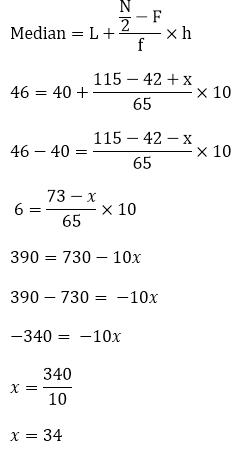Given N = 230

12 + 30 + 34 + 65 + y + 25 + 18 = 230

184 + y = 230

Y = 230 - 184

Y = 46 (ii)

Class interval
Mid value x
Frequency f
Fx

10 -20
15
12
180

20 - 30
25
30
750

30 - 40
35
34
1190

40 - 50
45
65
2925

50 - 60
55
46
2530

60 - 70
65
25
1625

70 - 80
75
18
1350

N = 230
Σfx = 10550= 45.87

Question: 12

If the median of the following frequency distribution is 28.5 find the missing frequencies:

Class interval:
0 - 10
10 – 20
20 - 30
30 - 40
40 - 50
50 - 60
Total

Frequency:
5
f1
20
15
f2
5
60

Solution:

Class interval
Frequency
Cumulative frequency

0 – 10
5
5

10 – 20
f1
5 + f1 (F)

20 – 30
20 (f)
25 + f1

30 – 40
15
40 + f1

40 – 50
f2
40 + f1 + f2

N = 60

Given Median = 28.5 Then, median class = 20 – 3017 = 25 - f1

f1 = 25 - 17 = 8

Given Sum of frequencies = 60

5 + f1+ 20 + 15 + f2 + 5 = 60

5 + 8 + 20 + 15 + f2 + 5 = 60

f2 = 7 f1 = 8 and f2 = 7

Question: 13

The median of the following data is 525. Find the missing frequency, if it is given that there are 100 observations in the data.

Class interval
Frequency
Class interval
Frequency

0 – 100
2
500 – 600
20

100 – 200
5
600 – 700
f2

200 – 300
f1
700 – 800
9

300 – 400
12
800 – 900
7

400 – 500
17
900 – 1000
4

Solution:

Class interval
Frequency
Cumulative frequency

0 – 100
2
2

100 – 200
5
7

200 – 300
f1
7 + f1

300 – 400
12
19 + f1

400 – 500
17
36 + f­1 (F)

500 – 600
20 (f)
56 + f1

600 – 700
f2
56 + f1 + f2

700 – 800
9
65 + f1 + f2

800 – 900
7
72 + f1 + f2

900 – 1000
4
76 + f1 + f2

N = 100

Given Median = 525 Then, median class = 500 - 600 L = 500, f = 20, F = 36 + f1, h = 600- 500 = 100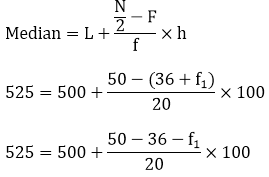25 = (14 - f1) x 5

5 = 14 – f1

f1 = 14 – 5

f1 = 9

Given Sum of frequencies = 100

2 + 5 + f1 + 12 + 17 + 20 + f2 + 9 + 7 + 4 = 100

2 + 5 + 9 + 12 + 17 + 20 + f2 + 9 + 7 + 4 = 100

85 + f2 = 100

f2 = 100 – 85 = 15

f1 = 9 and f2 = 15

Question: 14

If the median of the following data is 32.5, find the missing frequencies.

Class interval:
0 - 10
10 – 20
20 - 30
30 - 40
40 - 50
50 - 60
60 -70
Total

Frequency:
f1
5
9
12
f2
3
2
40

Solution:

Class interval
Frequency
Cumulative frequency

0 – 10
f1
f1

10 – 20
5
5 + f1

20 – 30
9
14 + f1

30 – 40
12 (f)
26 + f1

40 – 50
f2
26 + f1 + f2

50 – 60
3
29 + f1 + f2

60 – 70
2
31 + f1 + f2

N = 40

Given Median = 32.5. The median class = 90 – 40 L = 30, h = 40 – 30 = 10, f = 12, F = 14 + f1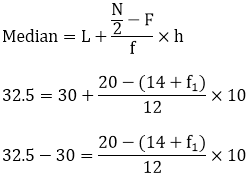2.5 (12) = (6 - f1) * 10

30 = (6 - f1) * 10

3 = 6 - f1

f1 = 6 - 3

f1 = 3

Given Sum of frequencies = 40

f1 + 5 + 9 + 12 + f2 + 3 + 2 = 40

3 + 5 + 9 + 12 + f2 + 3 + 2 = 40

34 + f2 = 40

f2 = 40 - 34

= 6

f1 = 3 and f2 = 6

Question: 15

Compute the median for each of the following data

(i)

(ii)

Marks
No of students
Marks
No of students

Less than 10
0
More than 80
150

Less than 30
10
More than 90
141

Less than 50
25
More than 100
124

Less than 70
43
More than 110
105

Less than 90
65
More than 120
60

Less than 110
87
More than 130
27

Less than 130
96
More than 140
12

Less than 150
100
More than 150
0

Solution:(i)

Marks
No of students
Class interval
Frequency
Cumulative frequency

Less than 10
0
0 – 10
0
0

Less than 30
10
10 – 30
10
10

Less than 50
25
30 ­– 50
15
25

Less than 70
43
50 – 70
18
43 (F)

Less than 90
65
70 – 90
22 (f)
65

Less than 110
87
90 – 110
22
87

Less than 130
96
110 – 130
9
96

Less than 150
100
130 – 150
4
100

N = 100

We have N = 100 N/2 = 100/2 = 50. The cumulative frequency just greater than N/2 is 65 then median class is 70 – 90 such that L = 70, h = 90 – 70 = 20, f = 22, F = 43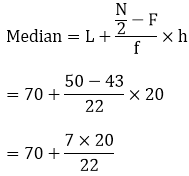= 70 + 6.36

= 76.36

(ii)

Marks
No of students
Class interval
Frequency
Cumulative frequency

More than 80
150
80 - 90
9
9

More than 90
141
90 - 100
17
26

More than 100
124
100 - 110
19
45 (F)

More than 110
105
110 - 120
45 (f)
90

More than 120
60
120 – 130
33
123

More than 130
27
130 – 140
15
138

More than 140
12
140 – 150
12
150

More than 150
0
150 – 160
0
150

N  = 150

We have N = 150 N/ 2 = 150/ 2 = 75.

The cumulative frequency just more than N/ 2 is 90 then the median class is 110 – 120 such that L = 70,

h = 120 – 110 = 10, f = 45, F = 45= 110 + 6.67

= 116.67

Question: 16

A survey regarding the height (in cm) of 51 girls of class X of a school was conducted and the following data was obtained:

Height in cm
No of girls

Less than 140
4

Less than 145
11

Less than 150
29

Less than 155
40

Less than 160
46

Less than 165
51

Find the median height.

Solution:

To calculate the median height, we need to find the class intervals and their corresponding frequencies. The given distribution being of the less than type, 140, 145, 150, 155, 160, 165 give the upper limits of the corresponding class intervals. So, the classes should be below 140, 140-145, 145-150, 150-155, 155-160, 160-165. Observe that from the given distribution, we find that there are 4 girls with height less than 140, i.e. the frequency of class interval below 140 is 4. Now, there are 11 girls with heights less than 145 and 4 girls with height less than 140. Therefore, the number of girls with height in the interval 140 - 145 is 11 - 4 = 7. Similarly, the frequency of 145 – 150 is 29 – 11 = 18, for 150 – 155, it is 40 – 29 = 11, and so on. So, our frequency distribution table with given cumulative frequencies becomes:

Class interval
frequency
Cumulative frequency

Below 140
4
4

140 - 145
7
11

145 - 150
18
29

150 - 155
11
40

155 - 160
6
46

160 - 165
5
51

Now n = 51. S0, n/ 2 = 51/ 2 =25.5 this observation lies in the class 145 – 150 Then, L (the lower limit) = 145 cf (the cumulative frequency of the class preceding 145 – 150) = 11 f (the frequency of the median class 145 - 150) = 18 h (the class size) = 5 Using the formula,We have Median = 145 + 72.5/ 18 = 149.03 So, the median height of the girls is 149.03 cm This means that the height of about 50% of the girls in less than this height, and 50% are taller than this height.

Question: 17

A life insurance agent found the following data for distribution of ages of 100 policy holders. Calculate the median age, if policies are only given to persons having age 18 years onwards but less than 60 years.

Age in years
Number of policy holders

Below 20
2

Below 25
6

Below 30
24

Below 35
45

Below 40
78

Below 45
89

Below 50
92

Below 55
98

Below 60
100

Solution:

Here class width is not same. There is no need to adjust the frequencies according to class interval. Now given frequencies table is less type represented with upper class limits. As policies were given only to persons having age 18 years onwards but less than 60 years we can define class intervals with their respective cumulative frequency as below.

Age (in years)
Number of policy holders fi
Cumulative frequency (cf)

18 – 20
2
2

20 – 25
6 – 2 = 4
6

25 – 30
24 – 6 = 18
24

30 – 35
45 – 24 = 21
45

35 – 40
78 – 45 = 33
78

40 – 45
89 – 78 = 11
89

45 – 50
92 – 89 = 3
92

50 – 55
98 – 92 = 6
98

55 – 60
100 – 98 = 2
100

Total

Now from table we may observe that n = 100 Cumulative frequency (cf) just greater than n/ 2 (i.e. 100/ 2 = 50) is 78 belonging to interval 35 – 40 So median class = 35 – 40

Lower limit (l) of median class = 35 Class size (h) = 5 Frequency (f) of median class = 33 Cumulative frequency (cf) of class preceding median class = 45So median age is 35.76 years

Question: 18

The lengths of 40 leaves of a plant are measured correct to the nearest millimeter, and the data obtained is represented in the following table:

Length (in mm)
No of leaves

118 – 126
3

127 – 135
5

136 – 144
9

145 – 153
12

154 – 162
5

163 – 171
4

172 – 180
2

Find the mean length of life

Solution:

The given data is not having continuous class intervals. We can observe the difference between two class intervals is 1. So we have to add and subtract 1/2 = 0.5 to upper class limits and lower class limits Now continuous class intervals with respective cumulative frequencies can be represented as below:

Length (in mm)
Number of leaves fi
Cumulative frequency (cf)

117.5 – 126.5
3
3

126.5 – 135.5
5
8

135.5 – 144.5
9
17

144.5 – 153.5
12
29

153.5 – 162.5
5
34

162.5 – 171.5
4
38

171.5 – 180.5
2
40

From the table we may observe that cumulative frequency just greater then n/2 (i.e. 40/2 = 20) is 29, belongs to class interval 144.5 – 153.5

Median class = 144.5 – 153.5 Lower limit (l) = 144.5 Class size (h) = 9 Frequency (f) of median class = 12 Cumulative frequency (c f) of class preceding median class = 17= 144.5 + 9/4

= 146.75

So median length of leaves is 146.75 mm

Question: 19

An incomplete distribution is given as follows:

Variable:
0 - 10
10 – 20
20 -30
30 – 40
40 - 50
50 - 60
60 -70

Frequency:
10
20
?
40
?
25
15

You are given that the median value is 35 and sum is all the frequencies are 170. Using the median formula, fill up the missing frequencies

Solution:

Class interval
Frequency
Cumulative frequency

0 – 10
10
10

10 – 20
20
30

20 – 30
f1
30 + f1 (F)

30 – 40
40 (F)
70 + f1

40 – 50
f2
70 + f1 + f2

50 – 60
25
95 + f1 + f2

60 – 70
15
110 + f1 + f2

N = 170

Given Median = 35 Then median class = 30 – 40 L = 30, h = 40 – 30 = 10, f = 40, F = 30 + f1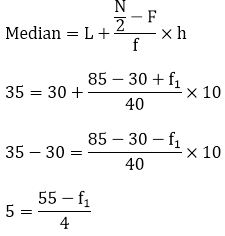20 = 55 - f1

f1 = 55 - 20 = 35

Given Sum of frequencies = 170

10 + 20 + fi + 40 + f2 + 25 + 15 = 170

10 + 20 + 35 + 40 + f2 + 25 + 15 = 170

f2 = 25, f1 = 35 and f2 =25
```### Course Features

• 728 Video Lectures
• Revision Notes
• Previous Year Papers
• Mind Map
• Study Planner
• NCERT Solutions
• Discussion Forum
• Test paper with Video Solution